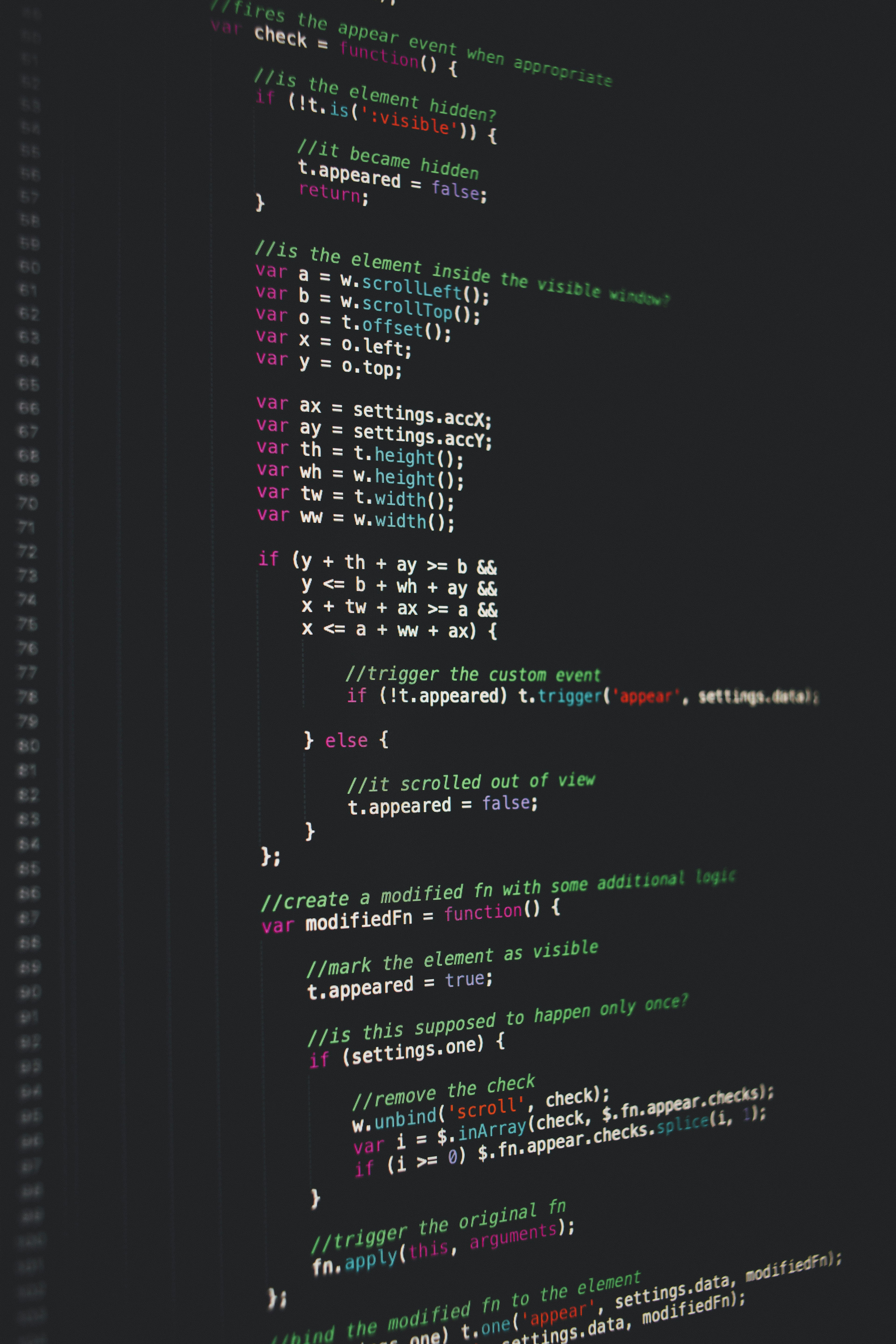Hackerrank Java Loops I Solution

# Hackerrank Java Loops I SolutionObjective
In this challenge, we're going to use loops to help us do some simple math.

Given an integer, , print its first  multiples. Each multiple  (where ) should be printed on a new line in the form: N x i = result.

Input Format

A single integer, .

Constraints

Output Format

Print  lines of output; each line  (where ) contains the  of  in the form:
N x i = result.

Sample Input

2


Sample Output

2 x 1 = 2
2 x 2 = 4
2 x 3 = 6
2 x 4 = 8
2 x 5 = 10
2 x 6 = 12
2 x 7 = 14
2 x 8 = 16
2 x 9 = 18
2 x 10 = 20


### Solution in java8

Approach 1.

import java.io.*;
import java.util.*;

public class Solution {

public static void main(String[] args) {
Scanner in = new Scanner(System.in);
int N = in.nextInt();

for( int i = 1; i <= 10; i++ ) {
System.out.println( N + " x " + i + " = " + N*i);
}
}
}

Approach 2.

import java.io.*;
import java.util.*;

public class Solution {

public static void main(String[] args) {
Scanner scanner = new Scanner(System.in);
int N = scanner.nextInt();

for (int i = 1; i <= 10; ++i) {
System.out.printf("%d x %d = %d\n", N, i, N * i);
}
}
}

Approach 3.

import java.io.*;
import java.util.*;

public class Solution {

public static void main(String[] args) {
Scanner sc = new Scanner(System.in);
int n = sc.nextInt();
for(int i = 1; i<=10; i++)
{
int result=n*i;
System.out.println(n+" x "+i+" = "+result);
}
}
}